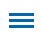One could easily argue that there is no more authentic math calculation than determining the area and perimeter. There are countless examples of 'real world' applications including:

• the necessity to calculate the perimeter of a room before deciding on the amount of carpet or crown molding you need to buy, or
• calculating the perimeter of your yard to better estimate the amount of grass seed or fertilizer required.

One of my all time favorite examples involves a mini golf course design. I remember fondly spreading out pieces of carpet and wooden two-by-fours on the floor of my fifth-grade classroom. My students then completed a performance assessment in which they had to calculate the area and perimeters of a handful of given hole designs, so they could order the correct amount of material. Finally, they could create their own design and have a friend attempt the calculations. You can use Mini Golf Measurements activity in Wixie to do the same activity.

Page 1 and 2 of the Mini Golf Measurements activity are designed to be reviewed by the teacher. The example shows two different methods of partitioning, which both produce the correct answer. Although partitioning may be a concept reserved for middle school students, the basic two- and three-part partitioning required in this activity adds to the authenticity of the task and involves manageable dimensions.

The final example, including triangles, may also be challenging. Students can solve the problem even though they don't know how to calculate the area of a triangle, if they can see that the two triangles are perfect halves and when combined equal a whole rectangle. It is conceptual thinking of this type that is being nurtured more and more in elementary math instruction.

In addition, the required 'explain your mathematical thinking' on this page will provide a snapshot as to how they went about determining the area of something other than a square or rectangle that they have yet to receive instruction on. The teacher may opt to modify or even delete any of the designs they feel may be too sophisticated for their students. Of course, I am all for leaving the design that involves triangles to see if someone can surprise you.

Instructional Concept: Geometric MeasurementLocation in Templates Library: Curriculum> Math > Measurement

Estimated Completion Time: 20-30 minutes

## Math Standards and Learning Goals

### Common Core Standards - Grade 3 Mathematics - Measurement and Data

3.MD.C.7.8 Multiply side lengths to find areas of rectangles with whole-number side lengths in the context of solving real world and mathematical problems, and represent whole-number products as rectangular areas in mathematical reasoning.

3.MD.D.8 - Solve real world and mathematical problems involving perimeters of polygons, including finding the perimeter given the side lengths, finding an unknown side length, and exhibiting rectangles with the same perimeter and different areas or with the same area and different perimeters.

### Virginia Standards of Learning - Mathematics - Measurement and Geometry

4.7 The student will solve practical problems that involve determining perimeter and area in U.S. Customary and metric units.

### Texas Essential Knowledge and Skills (TEKS) Geometry and measurement

3.6 The student applies mathematical process standards to analyze attributes of two-dimensional geometric figures to develop generalizations about their properties. The student is expected to:

(C) determine the area of rectangles with whole-number side lengths in problems using multiplication related to the number of rows times the number of unit squares in each row;
(D) decompose composite figures formed by rectangles into non-overlapping rectangles to determine the area of the original figure using the additive property of area.

3.7 The student applies mathematical process standards to select appropriate units, strategies, and tools to solve problems involving customary and metric measurement. The student is expected to:

(B) determine the perimeter of a polygon or a missing length when given perimeter and remaining side lengths in problems;

## Wixie Skills Review

The majority of this activity can be completed using a small-sized paint brush as if it is a pencil. I encourage teachers to direct students to use one color 'pencil' for calculating the perimeter and another for the area to help keep their work more organized and make it easier to assess/comprehend for the teacher.

The final page of the template directs students to create a mini golf hole design of their own. Students can fill squares on the grid with color using Wixie's Paint Bucket tool. The flag can be dragged to the desired location of the hole. Students can simply exchange devices to complete the calculations on a friend's design. They can also use the Team tool to invite another student to collaborate on their project.